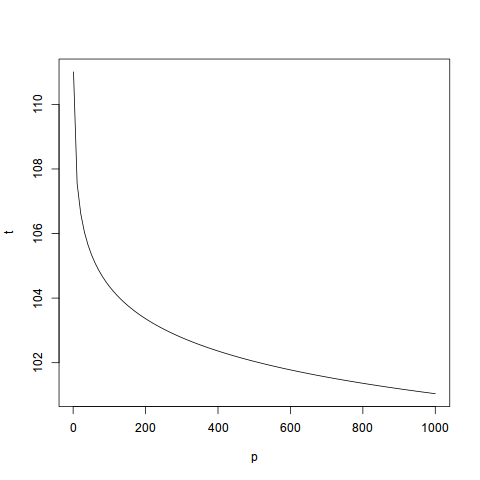Brute Forcing Intel x86 Instruction Correctness Via Unit Testing and an Intern Pool

# Brute Forcing Intel x86 Instruction Correctness Via Unit Testing and an Intern Pool

### m4b.github.io@gmail.com

To begin, let f(x) be a function from the number of bytes to the number of possible binary sequences for that number of bytes:

 f(x) = 2(8*x)     (1)

Since the largest Intel instruction can be 15 bytes, and the shortest 1 byte, we have exactly:

ω = 5.21265880699967e+33 =
 15 ∑ i=1
f(i)     (2)

possible instructions to test (2 to the power of 15 * 8, for example, is the number of different combinations of 0s and 1s for a 15-byte sequence, and hence the number of possible 15-byte instructions).

Let’s suppose half of them `#UD`. A more liberal estimate might be more appropriate, but half seems sufficient for our demonstration here.

Now, let’s suppose we have access to a large pool of interns, p, whom we task with committing a binary sequence, bi, of size i, where 1 ≤ i ≤ 15, and an expected value, ej, to a text file. To make things simple, let’s suppose ej is a constant number of bytes on disk, say 32.

Hence, the total number of bytes for this text file we require is therefore only:

 15 ∑ i=1
f(i i  (32+2)
2
(3)

where we add two (2) bytes for two (2) new lines in order to make the resulting text file more human readable.

Further, let us suppose we have already generated the binary sequences, and the new lines, which leaves only (ω * 32)/2 bytes to be committed to disk, by our pool of interns, p.

Let us further suppose that they only commit correct expected values (or their employment will be promptly terminated, and they will not receive a letter of recommendation).

Furthermore, as can be expected from our harsh treatment, they are exceptionally efficient at their task, and after each timestep, commit two (2) times as many expected values to disk.

Their rate of work1 , in bytes committed to disk, is therefore given by:

i(t) =  p  32
 t ∑ k=1
2(k−1)     (4)

where p is the number of interns and t is a continuous multiplier for some unit of time > 0.

As such, we wish to know what t equals when i(t) = (ω * 32)/2

Simple algebra2 gives us t as a function of i:

t(i) =
ln (
 i (32  p)
+ 1)
ln 2
(5)

For the sake of argument, let us suppose our summer internship program had a successful recruiting session, and we yielded four (4) interns, hence p = 4 and therefore:

t(ω  16)=
ln (
 ω * 16 32*4
+ 1)
ln 2
ln (
 8.34025409119947203e+34 128
+ 1)
0.693147180559945286
 75.5569565803551768 0.693147180559945286
= 109.005646563141141
(6)

Therefore, if our unit of time is a single day, which seems reasonable given the constraints above, then we can estimate that a pack of four (4) interns can finish writing a complete x86 instruction unit test suite for expected values to disk in approximately the duration of a 3 and 1/2 month summer internship.

Figure 1 illustrates the effect of p on t, when our pool of interns monitonically increases.Figure 1: Effect of number of interns p on t

As expected, adding more interns only marginally decreases t, with the “sweet spot” around one-hundred (100) interns.

However, for larger, more serious business enterprises, the extra six (6) days of extra business activity could be an actionable justification for the expenditure required in maintaining a larger intern pool.

In conclusion, contrary to previous efforts, we have demonstrated that a complete unit testing suite for the x86 instruction set is a tractable problem, given the right assumptions.

1
David 2015
2
Clarence 2015

This document was translated from LATEX by HEVEA.